# Part XVII Connecting the Dots Part 8

## The Folding of Space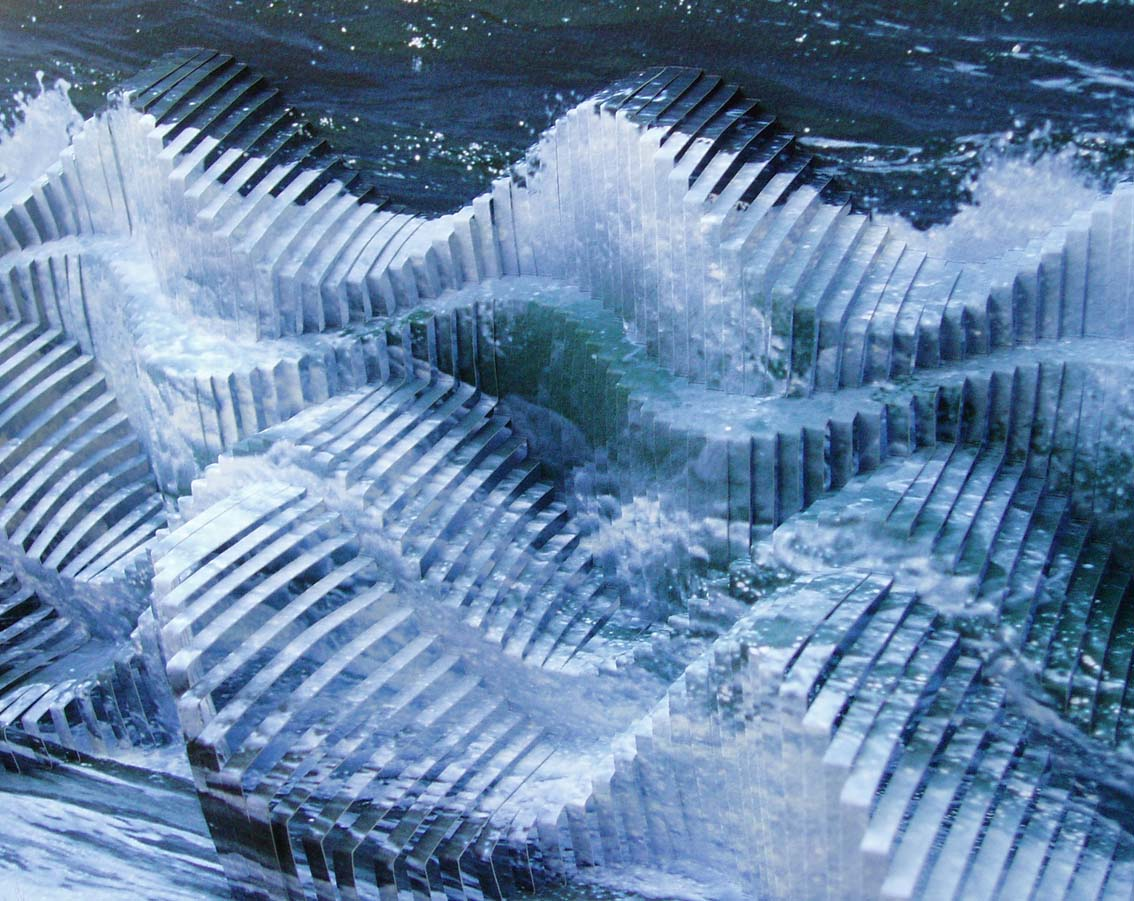Before we get into the wells of living waters dug by our forefathers, we want to circle back to the global structure of the Torah, the Alef-bet, and the universe.  This section of the series will be deeper than most and thus more profound.  The mathematics is not more complex, but perhaps a little more abstract and enlightening.

The total number of the 5 main elements—Words, Letters, Verses, Rows and Columns—in the Torah is 401,273 or 248,000/ Phi(φ).  The total number of the 3 main elements—Words, Letters, Verses—in the Torah is 390,625 or 58.  The Rows and Columns portion of these numbers is 10,648 or 223. As we will see later in this section, it is not coincidental that the Rows and Columns in the Torah are the cube of 22, as in the 22 letters of the Alef-bet. When the Tower of Truth is extended to 22 levels or 22 cubes, the total cumulative volume is 64,009 cubits or 40.001873 cubic cubits. Keep in the back of your mind that (40 – 22) = 18. There are exactly 187 chapters in the Torah, 40 of which are in the Second Book.  For anyone that has not been following this series from its inception, you can find the entire work here.  Combined into a single equation the relationship between the Torah’s main elements is: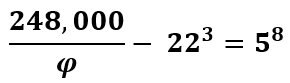We will not get into the meaning behind these individual numbers, as we have done so numerous times in this series already, though, we do want to point out that 248,000 is equivalent to (31 x 8000) or (31 x 203), the 20th cube in the 20 stacked cubes of the Tower of Truth. Moreover, EL (אל) is G-d and has the numerical value of 31, and there are 20 multiples of 31 in 620, the dimension of Keter of the same numerical value as Esrim, the Hebrew word for 20, and yes, EL (אל) has an ordinal value of 13, Echad, One, for a total gematria of 44, as in the 44 Chanukah candles and the regressive form of Ehyeh (אלף־הי־יוד־הי), but we are looking beyond gematria for the moment: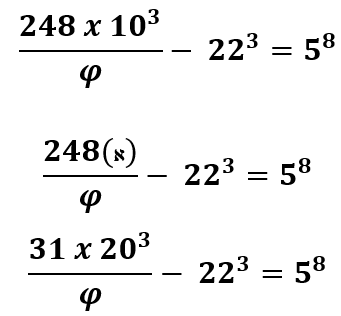In the last section we saw that Phi(φ) creates infinity, and that Phi(φ) creates harmonic order and efficiency.  Phi(φ) is the link between the physical field forces and the ethereal field forces.

Now we must ask: What came first, the spatial structure or the document? Black fire on White fire.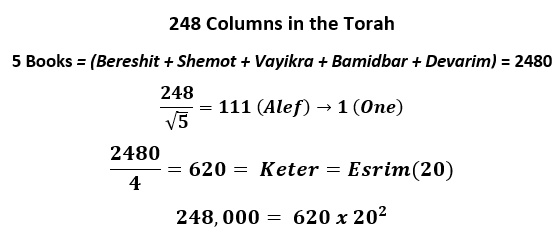Does Abraham (248) unfold 8 times like the Torah into Abraham(א), Isaac(י), Jacob(י) and Joseph(י)? There are 4 Patriarchs, 4 Archetypes, 4 Forces, 4 Movements, and 4 initials (א י י י) that sum to 31.

Is this also a model for the folding of space?

Abraham(248) had 8 sons in total.  Is this a model of the E8 Lattice supersymmetry and E8 Lie groups with 248 dimensions that are structured around the Phi(φ) proportion.  Zeir Anpin is a bundle of 6 dimensions, represented by the 6 directions of the simple cube and the 6 wells our forefathers dug to open the springs of living waters. There are 240 faces of a 6-d hypercube; might they connect with the 240 non-base dimensions of the E8 Lattice? To clarify, it has 8 base dimensions, or coordinates, and 240 non-base ones for a total of 248. Within that 248-dimensional E8 root lattice its E8 root system consist of, and is broken down into, groups of 8 vectors (roots) that conform to specific parameters, and 112 of those groups are integers.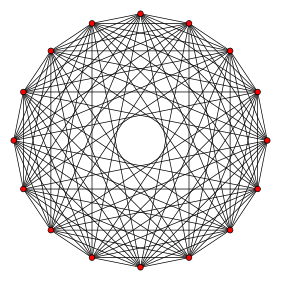Each point in the E8 Lattice is surrounded by 2160 8-orthoplexes and 17280 8-simplices. Each 8-orthoplex is a regular 8-polytope with 112 edges.You can see in the accompanying projection that the 8-orthoplex is designed the same way as the 231 Gates that Abraham described for us in his Sefer Yetzirah, the difference being that Abraham used the 22 Letters as vertices and the 8-orthoplex has 16.

Each point in it is surrounded by 2160 8-orthoplexes and 17280 8-simplices. Each 8-orthoplex is a regular 8-polytope with 16 vertices and 112 edges. Phi(φ) appears in the ratios of the quasi-crystals, and can also be seen in the eigenvalues of the eigenvector matrices within the E8 lie group. What is pictured is an E9 Lie group, the seed of infinite E8 with its eigenvalues, where Phi(φ) and Phi(φ2) can be seen throughout.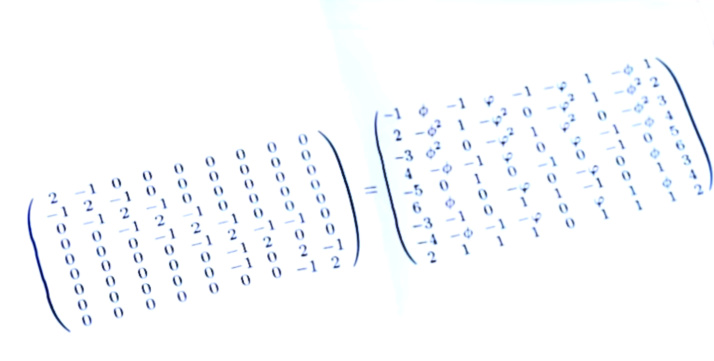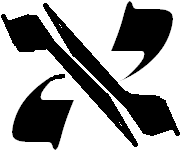There are 248 words in the Shema, imagine if each one, instead of representing a different dimension, actually connected to one.  That would be something! It is also something still far beyond our comprehension though. Fortunately, we have been given a path to comprehension. By aligning ourselves with the Plan of Potentiality, the path between the ethereal forces, the Vav(ו) in the YHVH (יהוה). When the Vav(ו) splits in two, like in the Alef(א), the path through Zeir Anpin to Binah is open, the YHVH (יהוה) goes from 26 to 32, like the Alef(א), and you can connect with all the tzaddikim and all the elevated souls who have been there before you. You can receive pure visions in this state.

It will be easy to reach the proper state when the 112 Triplets are aligned.  Peter has just pointed out some wonderful new insights about them.  Given all the Phi(φ) connections to 18, 18o, and chai, life, of numerical value 18, he started with the 18th Prime number, which is 61.  The value 61 is the numerical value of Ani (אני), “I” in Hebrew, as in “I am your God.” Regarding portion Miketz, the Zohar 15:250 asks “Who is ‘I?’ Rabbi Shimon replies, “It is the Holy One, blessed be He, the sacred kingdom of heaven…Come and behold: ‘I’ is the government of this world and of the World to Come, and everything depends on it. Whoever clings to the ‘I’ has a portion in this world and the World to Come.” Rav Ashlag, of blessed memory goes on to explains that Rabbi Shimon was referring to the Nukva, the adjunct to the YHVH (יהוה) in our world.

Peter connected the 18th Prime Number, 61, with 610, the value of the 3rd line/iteration of the Upper 42-Letter Name. He then merged it with the first line of the Lower 42-Letter Name of numerical value 506. The two Names conjoined at this transition point sum to (610 + 506) = 1116 + 2 (kolel) = 1118. In the same way that Keter and Malkut do, (620 + 496 = 1116), and also the first two words of the Torah, Bereshit and Bara (913 + 203 = 1116). With the kolel, all equal 1118 in the same way and in the same functional meaning.

This is, of course, is significant because the 15 Triplets of the first verse of the Shema, one of the 4 Essential Elements of the universe that comprise the 112 Triplets has the numerical value 1118 and it too is the combination of the full Upper Name of 42 of numerical value 708 and kadosh Holy (410).  We are reminded of the connection between 3 of the 4 Essential Elements—the Shema, the Torah’s first verse, and the Lower 42-Lettter Name—and both the pivotal calendar year, 3760 HC, and the 248 dimensions: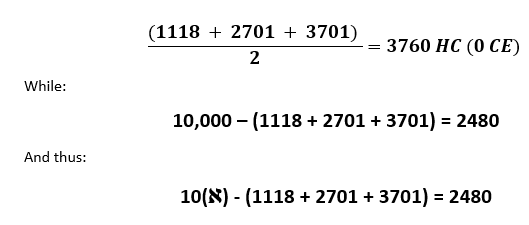## A Hypothetical

We have revealed that the Hebrew alphabet is derived from an exponential scale based on the number 1.313 raised to the ordinal value of each letter. Later in this section we are going to reveal much more about that. Meanwhile, if we instead use an exponential scale whereby each ordinal value is raised to 1.313, instead of the other way around, some very interesting and germane results pop out. We will call this scale hypothetical for now.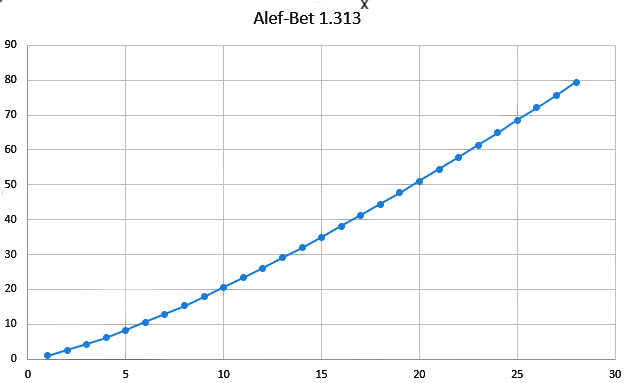We can see the curves are quite different with the top (hypothetical) one being somewhat linear and obviously their result values for the Hebrew letters would be quite different as well. Alef(א) in the hypothetic model has a value of exactly 1 and Bet(ב) has a value of 2.48… and Gimmel (ג), 4.23… as in Phi(φ3).

If we subtract the hypothetical values from the results of the exponential scale the 18th letter, Tzadi (צ), of numerical value 90, has a difference of 90.03242… Keep in mind that the sum of the digits in mysterious repetitive 18-digit Fibonacci sequence (173553719826446289) is 90, the numerical value of water. These connections are not coincidental, as will be expanded upon below. Perhaps neither is the fact that the value of Tzadi (צ) on the hypothetical scale is 44.480, reminiscent of 2448 HC.

Nor is it coincidental that the difference in the value of the two scales for the 21st letter, Shin (ש) is 250.0207… with 207 being the gematria of the word for “Light,” the 25th word in the Torah. The Hebrew word for candle, ner, has a value of 250, representing the two enlarged letters in the Torah (נר). The connect to the 21st letter are more profound as will be explained shortly.

Of probable significance is that the letter Lamed(ל) on both scales is approximately 26, as in the YHVH (יהוה), and they are only off from each other by .133358

Finally, the 26th letter, Pe(ף) sofit, has a value of 72.08… in the hypothetical scale, and the difference in the two scales is 1116.09424….. Given all the pairs that sum to 1116 within the 4 Essential Elements comprised of the 112 Triplets, including the 72 Triplets, this connection is not coincidental either. It is interesting that Alef (אלפ) in this scale is (1 + 26 + 72).  Further study will elucidate the deeper connection between these two star-crossed scales.

## Phi and the Secret of the Pyramid

Peter then went on to connect the Pyramid’s slope angle (51.84o) at the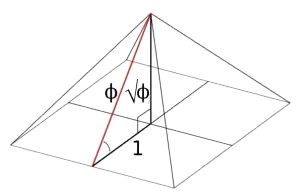center of its faces to the 4th of the 4 Essential Elements, the 72 Triplets in that 5184 = 722. That is probably no coincidence, as the slope angle of the Pyramid is 42o at its corners, and the face to face edge angle is 112o, and the apex angle is 76o, as in the ordinal value of the Torah first word.  Of course, they all connect to Phi(φ) in that there is a Phi(φ) triangle that Kepler came upon in his studies of the ellipses of the planets.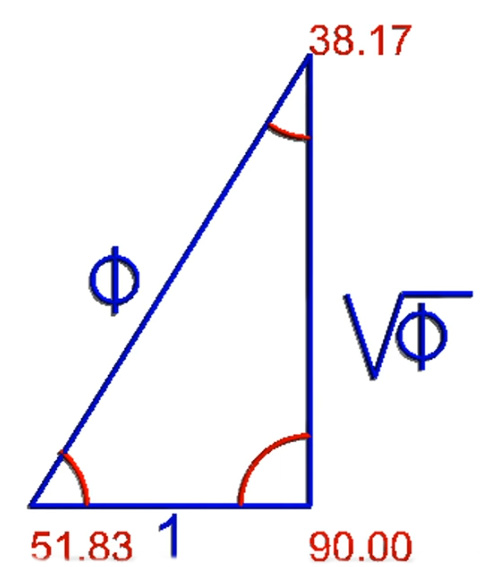Even though the first mention in history of Phi(φ) was by Euclides in about 300 BCE, many have suspected the Pyramid was based on Phi(φ) proportions. As we have revealed there is a lot more than that though.  Peter highlighted the Phi(φ) angles to the Pyramid’s slope angle (51.84o) at the center of its faces and to the 4th of the 4 Essential Elements, the 72 Triplets in that 5184 = 722. We want to go further and explain that the number 72 is directly connected to Phi(φ) and the 27.5” cubit used to build the pyramid.

First of all, 72/27.5 = φ2. This obviously has tremendous ramifications for the Holy Temple to be built to the same 27.5” cubit. Next, 72/φ = (72 – 27.5). Then (72 – 27.5)/2 = 22.25, as in the Phi(φ) 222.5o angle, and by simple math 5(72 – 27.5) = 222.5.  Finally, 72/27.5 = (1 – 1/ φ).

There can be no doubt the Pyramid was built with the numbers 72, 27.5 and the Phi(φ) proportion in mind.  Internally, one enters the Pyramid from a height of 55’ or 2 x 27.5, then they pass through a passage 42” wide before encountering the descending passage, which is  26.18 o or φ2 degrees steep, and then ascending through the grand gallery, sloped at 26o, like the Tetragrammaton(יהוה). Of significance, far beyond the Pyramid is that 26φ = 42.

##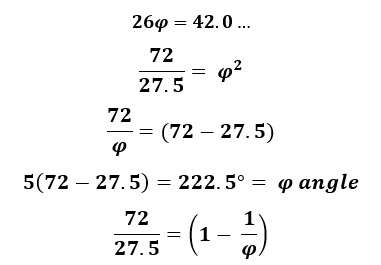Secrets of the 20th Cube

Now, we will see where the 20 stacked cubes of the Tower of Truth at the core of the Pyramid comes to play in its design once again.  The Tower and the Pyramid are 210 levels and 210 cubits tall, and 20 x 27.5(1 – 1/φ) = 210.0. It is off from 210.09826 by only .008%.

Then we know the 4 bases of the Pyramid were purposely built to 4 slightly different dimensions and the West base is within a fraction of an inch of 755.51 feet. As it turns out, 20 x 27.5(2 – √φ) = 755.5148.  Keep in mind that Caf(כ) of numerical value 20 is the letter that is not only in the middle of the exponential curve of the alef-bet, but also stands out as the one precisely on curve.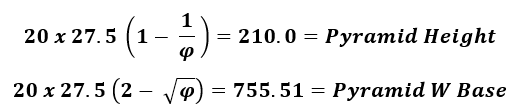The letter Caf(כ) is the 11th letter in the Alef-bet and (20 x 27.5)/11 = 50, as in the 50-cubit height of the gates in the Holy Temple and the 50 Gates of Binah.

The 20th Cube in the Tower of Truth has a volume of 8000 cubits3, or 203, which matches the value of the 64 Triplet 8 x 8 square base within the 72 Triplets. The value of the separate first 8 Triplet row is 1143. Considering the first 8 words in the Torah have 33 letters which comprise the 11 Triplets, it is interesting that 1143 beaks down to (1110 + 33) or put another way, (10 X Alef(אלף) + 33).  This is in the same manner that the 1118 value of the Shema’s 15 Triplets breaks down to (1110 + 8) or (10 X Alef(אלף) + 8).

11 Triplets: 8 Words = 33 Letters

15 Triplets: 1118 = (1110 + 8) = (111 x 10) + 8 = 10(אלף) + 8

8 Triplets: 1143 = (1110 + 33) = (111 x 10) + 33 = 10(אלף) + 33

64 Triplets: 8000 = 1000 x 8 = 8(אלף)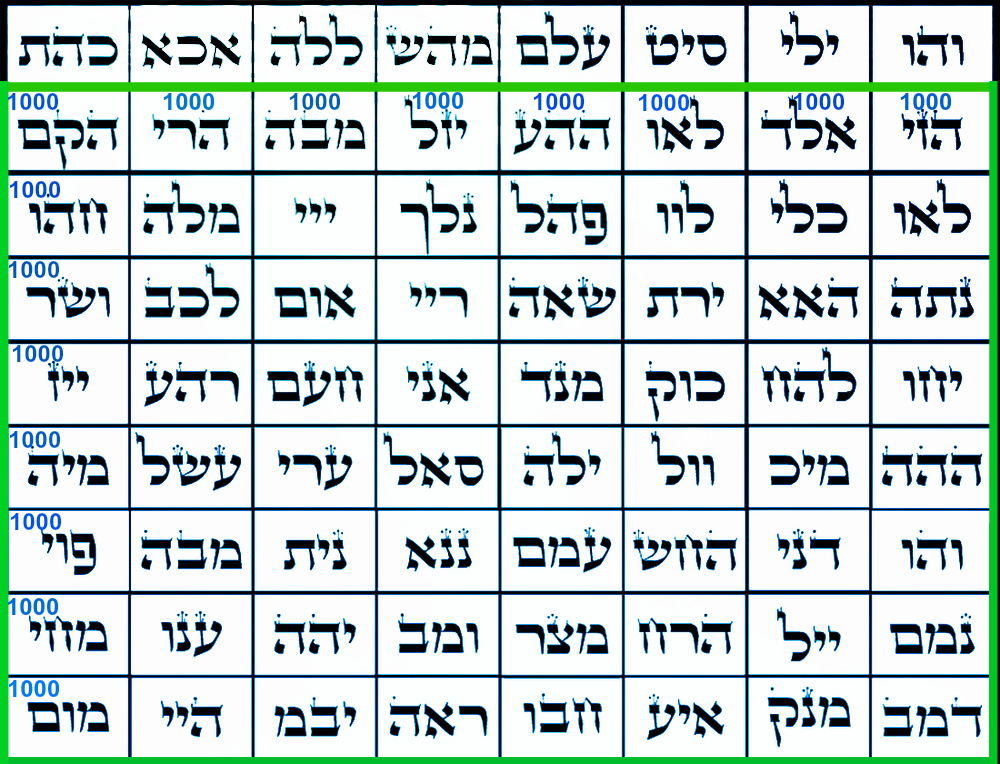Nonetheless, when we add 1143 of the 8 Triplets to 1118 of the Shema’s 15 Triplets we get (1143 + 1118) = 2261.  This is exactly 7000 less than, and reminiscent of, the 21st cube (213) which totals 9261.  It is also reminiscent of the sum of the Truth Cubes from 13 to 213, which is 53361.  That sum itself, 53361 is reminiscent of the sum of the 12 permutations of the YHVH in small gematria (i.e. YHVH = 1565), which is 53328, a difference of 33 from 53361.

Nevertheless, it was the equation 9261/53361 = 213/(Σ(13213) = .173553719… that was so mysterious and intriguing, and therefore it is auspicious that 2261/53361 = .42371… or .424, Moshiach Ben David.  Auspicious and intriguing.

it is interesting that 1143 beaks down to (1110 + 33) = (10 X Alef(אלף) + 33). We are about to see just how interesting.

## From Darkness to MoshiachThere is another number that has a powerful and special connection with Phi(φ), and truthfully there are not many of them except the powers of Phi(φ) like 5778 as φ18.  This special number is the numerical value of Moshiach Ben David (424).  Not only does 424φ2 = 1110.046 or 10 X Alef(אלף), but we also have 424/φ = 333.3281842 or 333.33. If you recall, 333 is the gematria for darkness, H’chosech (הַחשֶׁךְ), and as we saw in the equation provided by the 4th verse of the Torah, “And God saw that the light was good; and God divided the light from the darkness (וַיַּרְא אֱלֹהִים אֶת הָאוֹר כִּי טוֹב וַיַּבְדֵּל אֱלֹהִים בֵּין הָאוֹר וּבֵין הַחשֶׁךְ) of numerical value 1776, the two portions of “The Light (הָאוֹר)” each of numerical value of 212 in Moshiach Ben David (424) form the proportion 424/333 = 1.273. This is the proportion of inscribing a circle within a square, bounding the darkness with Light, and it is approximately φ or 1.272019…., which is the reason 424/φ = 333.33 and not 333. Please note that the word for “The Light (הָאוֹר)” is the 4th word of the 4th verse of the Torah.

Notice also that the separation between 424 and 333 is 91, as in the unified (יאהדונהי) Names of YHVH (יהוה) + Adonai (אדני) that bridge our world to the World to Come.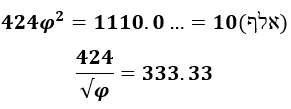If Alef(אלף) = 1000, and “the darkness” = (424/φ) = 333.33, what is (אלף424/φ), which equals (1000 – 333.33)? It is 666.66. What follows Alef(אלף) in the Alef-Bet, and what letter heads up the Torah? Bet(בית), of numerical value 412. What is Bet(בית) x Phi(φ)? (בית)φ = 412φ = 666.63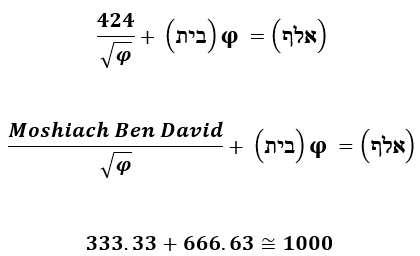## The Light and Phi

There is another association between “The Light (הָאוֹר)” and Phi(φ) and it relates to Pi as well, and to the mysterious repetitive Fibonacci sequence from every 4th number in it, 17355371.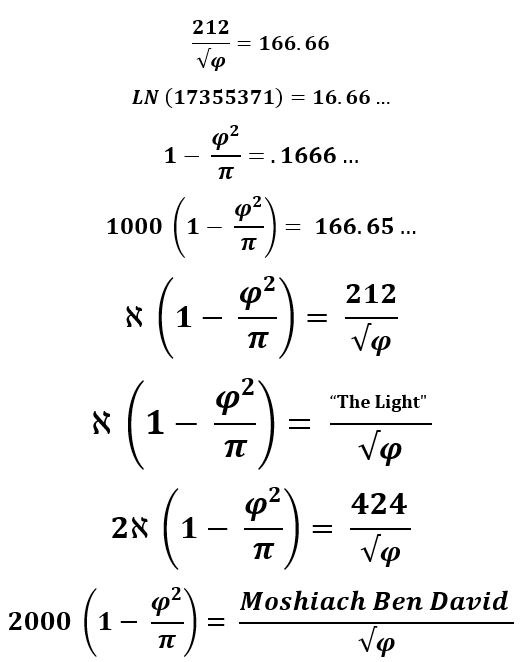According to chazal, the word for alefs is alafim (אלפים) and alpayim (אלפים), meaning thousands, and specifically signifying 2000, as in the verse Notzer Chesed L’Alafim from the 13 Attributes.  This refers to 2000 generations or iterations of refining the light from the darkness, good from evil.While 212 is the value of “The Light(הָאוֹר), 2120 is our assumed measure for the length of the  sides of the 10-cubit tall Chiel within the Temple Mount, which gave us the total volume of the Holy Temple and Mount as a cube of 424 x 424 x 424 cubits, the Moshiach Ben David Cube.

Why are cubes so integral to these formulas? Is Phi cubed(φ3) = 4.2360 related to 42.4, the Moshiach Ben David number? It does lead to φ3/φ = 3.330….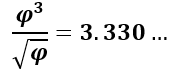Is this why there are 3330 years between the reception of the Torah at Mt Sinai in 2448 HC and the event horizon in 5778 HC, 50 jubilee years, analogous to φ18?

Why is Phi cubed(φ3) or 4.2360… integral to the spiraling vortexes of magnetic radiation? Why the Phi(φ) angel (137.5o) continually proceeds around a 360o circle, it will generate the Phi(φ) spiral.  On a single rotation cycle there will be two turn of (137.5o) and a remainder of (360o2(137.5o)) = 85o, and 360/85 = φ3. Those two first turns (137.5o) and (137.5o) equal 275, as in our 27.5” cubit. So a cubit is defined as 1” for every 10o in two(2) Phi(137.5o) turns. You could not use that physically unless you built a circle with a 57.295779” radius, but it is significant metaphysically. The connection to Phi(φ)  and Pi through the circle is included in every cubit measured out and used in Holy construction, or divinely inspired construction.

##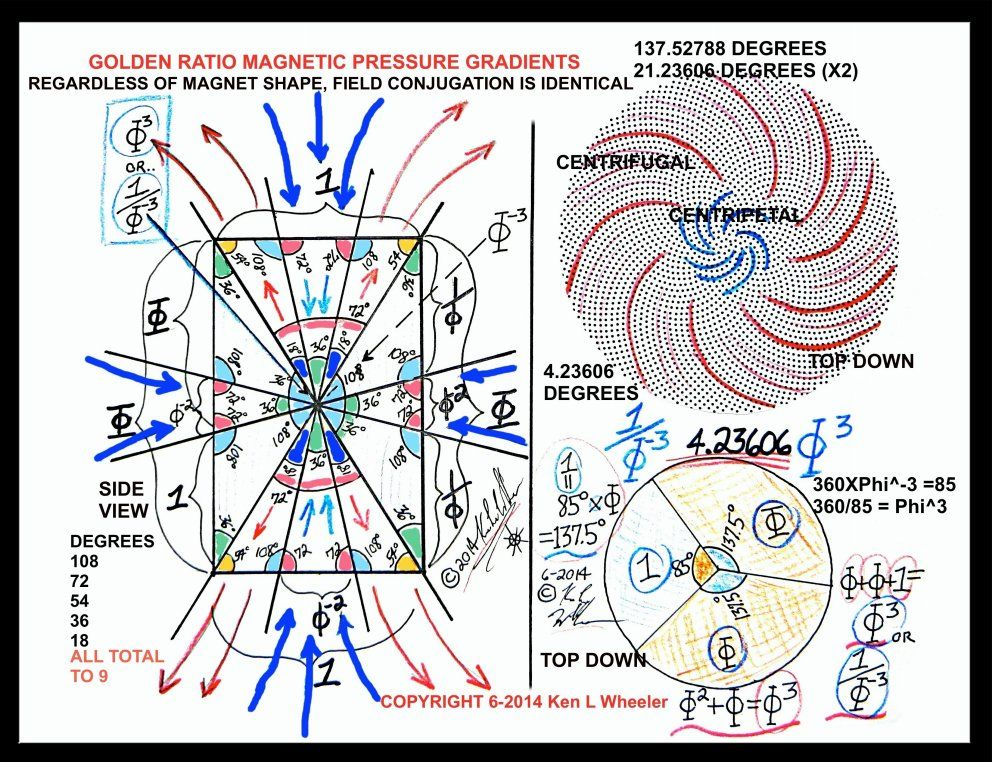Fibonacci and 72

When the 18 mysterious number sequence (173553719826446289) derived from Phi(φ) and independently from the 21st cube (213) is split into 9 pairs (1+7, 3+5, 5+3, 7+1, 9+8, 2+6, 4+4, 6+2, 8+9) and we reduce them to small gematria, we get (8+8+8+8+8+8+8+8+8) = 72.  Keep in mind that to get these 18 repetitive numbers, we took every 4th Fibonacci number, meaning the sequence repeats every (4 x 18) or 72 Fibonacci numbers. That is the beauty of a coherent design Created in a mind that can see infinite symmetry and how all things are connected.

## Waves, a Steering Force from Beyond

This may be abstract, but Nikola Tesla is often quoted as saying, “If you want to find the secrets of the universe, think in terms of energy, frequency and vibration.” Traditionally, we think of the Energy equation as (Planck’s Constant x the Speed of Light)/Wavelength, but that does not seem to fit Tesla’s concept, and it is no secret either, unless it is one day found to be false, of course, as most of our scientific formulas will be in time, replaced by a better one given more data about our universe, as is logical and as was suggested by Einstein himself later in his life.

Could Tesla have been referring to the Alef(אלף)? The Alef(א) itself could represent Energy and the Lamed(ל) frequency and the Pe(פ) vibration, alternatively the representations of the Lamed(ל) and Pe(פ) may be reversed.  Energy is the result of the combined: wave length, which is the measure of the wave from crest to crest; frequency, which is the number of full s-shaped waves or cycles within a unit of time; and vibration would be amplitude, or height of the wave at its crest off its mid-line.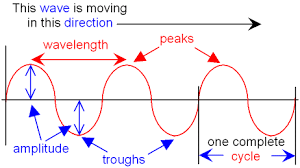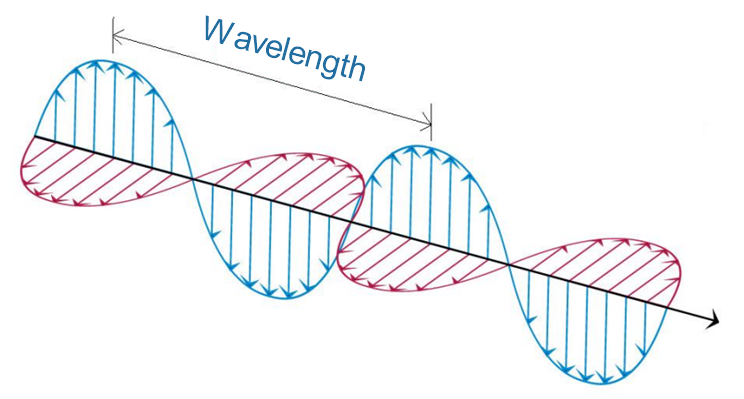The Energy they are talking about is electromagnetic radiation, including light, radio, microwaves, and gamma rays alike.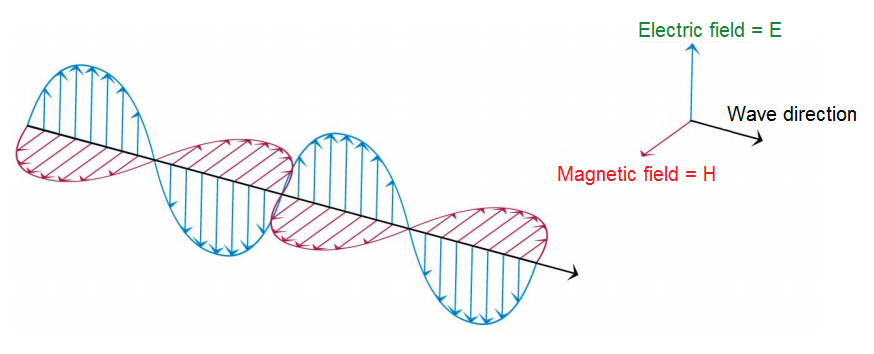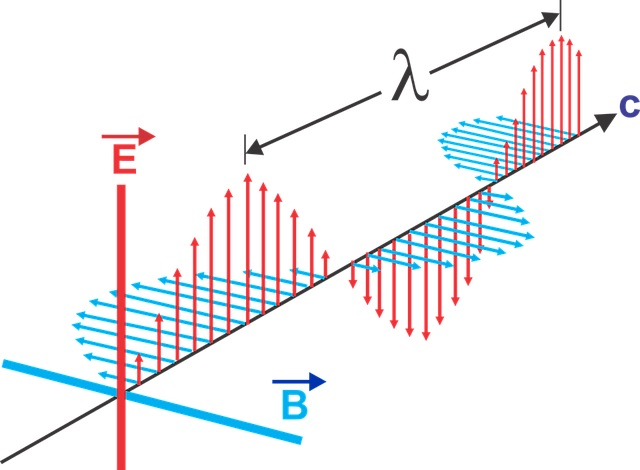Energy is based on waves, and waves are based on ether; in other words, something metaphysical rather than physical.  Yes, that is odd for scientists who abhor the notion of an ether, preferring to think that even if the vast majority of what is going on in the universe is unknown, it will still conform to the physics that we already imagine as correct.

A wave is an energy transport phenomenon that transports energy along a medium without transporting matter, and in most cases in the universe that medium is unknown.  Yes, a wave can propagate through water, and sound waves can glide through the molecules in the air, and waves can vibrate through solid matter, but ask yourself: how much matter is there in the universe and how solid is that matter? Exactly.

Also, even when it is surging through a known medium, why does it? What keeps it straight and narrow? Why does it not veer off track? If it did, light would never reach us.  It is as if waves are not really there and only exist on paper to help make the theories work better.  On the other hand, we can see them in water and measure their impact, but what are they? Is the wave you see rippling over the surface of the water actually moving or has an action, like a tossed stone, caused a special chain reaction in the water” The water molecules are not going anywhere, besides up and down, so what it a wave?

Waves, as scientists conceive them, do not even fit all their theories, not even on paper, let alone in reality. There are some newer and possibly better theories that are quite interesting but not for today’s discussion. First, let us understand the simpler aspects of a wave.A disturbance within a medium, even the ether, subsequently travels along its midpoint in the wave field, never straying further than its amplitude in either direction, swinging back and forth like a perpetual pendulum, advancing cycle after cycle, sometimes for billions of years. This possibly goes on forever as and the energy just gets transferred to a new wave with energy never being lost.

Is a wave a perturbation in a field? Is the midline akin to the Plane of Potentiality, the Plane of Inertia, we saw between the two equal and opposite primal forces, divergence and divergence (curl/sink)?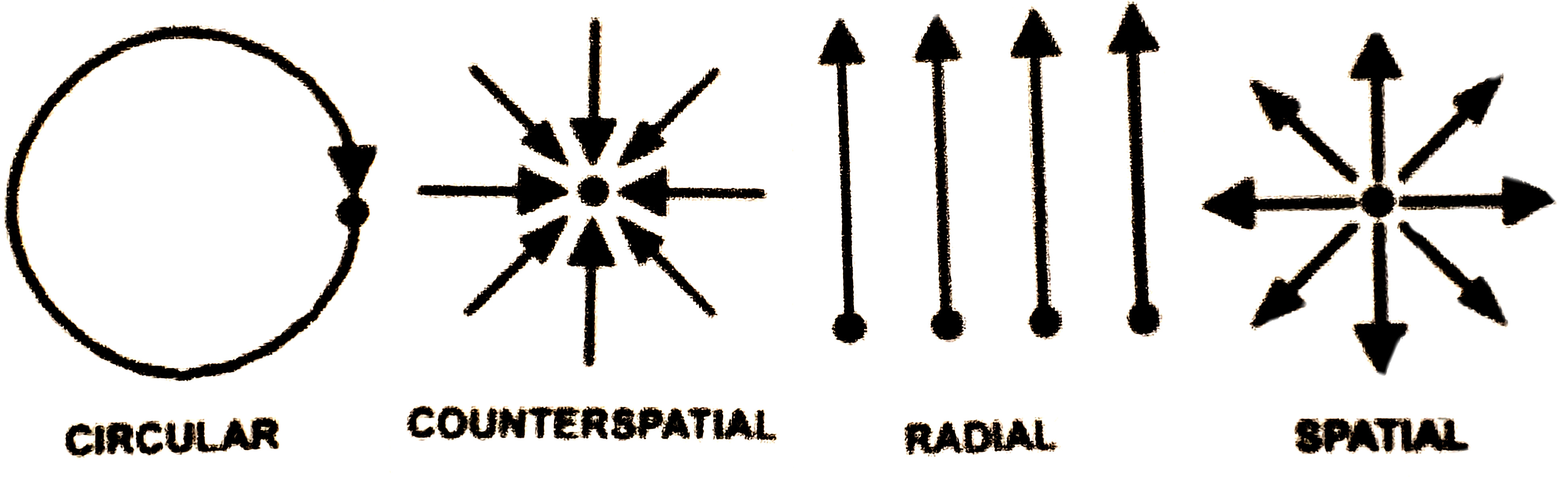Amplitude (the height of the waves) measures how much energy is being transported by the wave. The larger the amplitude, the more energy a wave has. Think of it as the volume control on a stereo, which used to be called an amplifier. The amount of energy carried by a wave is related to the amplitude of the wave. A high energy wave is characterized by a high amplitude; a low energy wave is characterized by a low amplitude. The energy transported by a wave is directly proportional to the square of the amplitude of the wave. Amplitude seems to be important, as the measure of the perturbation.  It is conveying information about the energy level being passed on.

Energy increases as the wavelength decreases, which consequently causes the frequency to increase, which is why the wavelength is the critical non-fixed component of our current Energy equation (Planck’s Constant x the Speed of Light)/Wavelength.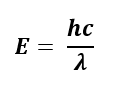How can the Alef(אלף) connect to the wave functions? We do not know and it is only a supposition at this point. We do know that the answer to the mysterious wave property of Energy must have its origins beyond the physical world and it is more likely to be an outcome of field interaction than anything else proposed so far. Nikola Tesla has rarely steered anyone wrong.

Whatever they are, waves provide the order to our physical world.

## The Fractal Alef-Bet

As an extremely important aside, the alef-bet exponential scale also displays a discernible wavelike pattern, though one a bit more fractal in nature until we look deeper when the real beauty unfolds.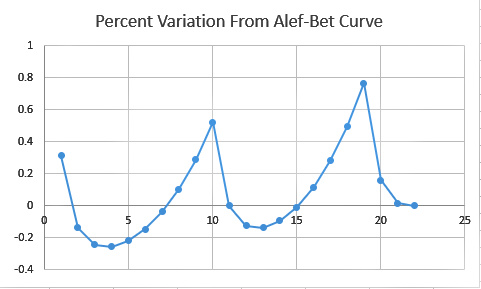This is a line graph without the curves being smoothed out, but you can already notice a nice curved repetitive pattern, growing fractally as the alef-bet progresses. Notice how that curve peaks at three points, at the Alef(א), the Yud(י) and the Koof(ק) or numerical values 1, 10, and 100 respectively in exponential progression, or geometrically by a multiple of 10. They total 111, as in Alef(אלף). While their ordinal values progress arithmetically by 9: 1, 10, 19. The value of the 28th letter would be 1000.

One further side note is that the amount the Koof(ק) is off exactly and percentagewise from the 1.313 exponential curve compared the same amounts that the Yud(י) is off is 244.8. This is most interesting because The Torah was given at Mt Sinai in the year 2448 HC and -1313 CE. How is that for pulling something out of the ether?

When we extend the graph to what would be the 28th letter at value 1000, we get the same extended fractal image of the percentage variations from the curve.And the slope between the fractally corresponding points is 1.6.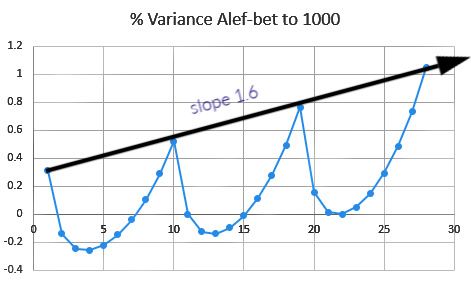What is most interesting is that that slope line is also the Alef(אלף) line, since the values 1, 10, and 100 and their corresponding letters Alef(א), the Yud(י) and the Koof(ק) are on it.

As we can see in the next graph where we flipped the variance curve along the Alef slope axis, that the curves are indeed symmetrical.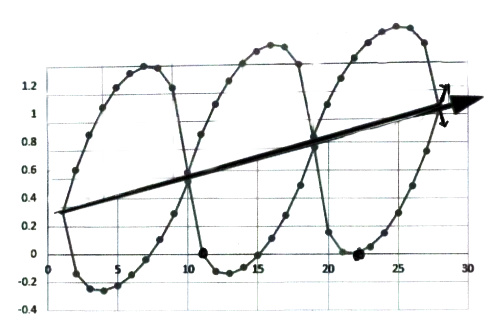We can also see that it is a sine wave or at least based on one. It is bottom half of an out of phase, or cancellation, wave sine wave where the two waves cancel each other out.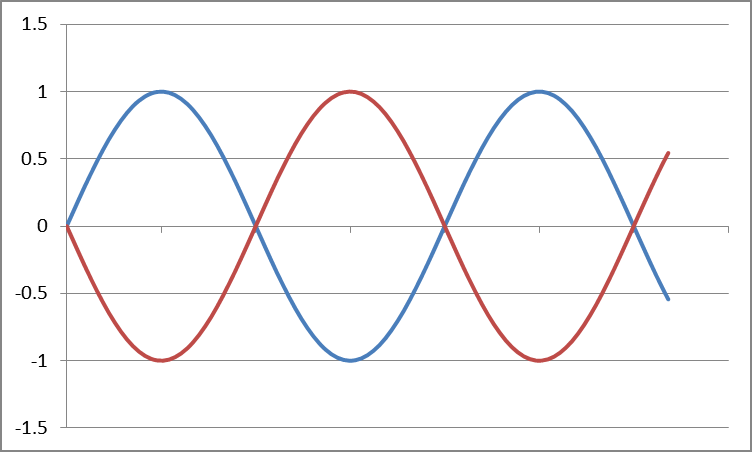It also conforms to the top half of a longitudinal wave.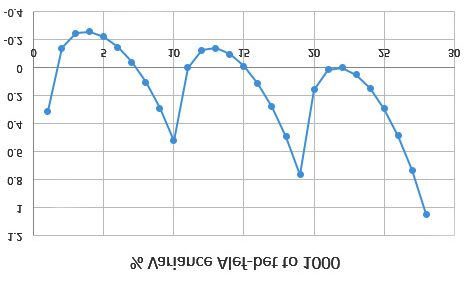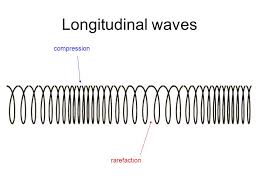## Find the function of these waves and find a trove of secrets.

If you find the function of these waves you will find a treasure trove of secrets and a slew of new information will be revealed. Its a simple formula complicated by the slope. There are actually two very similar waves symmetrical to the slope. The coordinate points are given by the ordinal values of the letters and the exponential Alef-bet equation (y = 1.313x)  Its a very different one for the longitudinal wave or slinky option.

We also need to ask what corresponds to the missing or hidden portions of the wave function? What is on the other side of the slope?Now, remember, the actual Alef-bet curve looks like this, not a straight-line axis, so this sine wave is actually symmetrical along the exponential curve.This should remove any doubts that the Alef-bet was meant to fit this curve based on 1.313.  So why this symmetry to the Alef(אלף) axis, whose slope is 1.6 or approximately Phi(φ)?

Then when we add the absolute value for all 27 percentage variances, they equal 6.1823 or approximately 1/Phi(φ) or .618033….  Moreover, the 21st letter, Shin (ש) has a variance off its nominative value (300) of 4.48033… or 1.70000… less than 1/Phi(φ). And since 17φ = 27.5, the cubit: 10varשφ= 27.5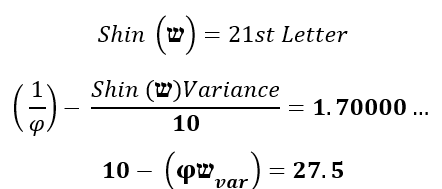One of the many treasures found within, and yet another relationship between Phi(φ) and the cubit, this time buried deep within the secrets of the alef-bet.

The Alef-bet begins with the letter Alef(אלף) of numerical value One(1), but its deviation from the curve is .313, making its exponential value 1.313 as the starting point for both the Hebrew alphabet and the Israelites at Sinai (1313 BCE). We explained at the outset of this series that the Hebrew letters in their pure form represented a vibrational range and not specific values. Now we understand that the range for each letter is a percentage variation from the curve conforming to a specific wave function with a specific slope.

That vibration range equals the amplitude. The formula tells us Amplitude = how much Energy is being transmitted by the wave.  Do certain letters transmit more energy than others? Is there an entire new science and understanding in this?As the full wave cycle intersects the Alef(אלף) axis at Alef(א) and Koof(ק) of ordinal values 1 and 19, the wavelength is (19 – 1) = 18. How interesting!

The number 18 comes up as a Phi(φ), comes up as our Alef-bet wavelength, as the mysterious repetitive Fibonacci sequence, as “Life,” and more. The square root of 18 is no less than 4.24264068…, or both 42-Letter Names, Moshiach Ben David(424), the Tetragrammaton(26), etc.. So what is the meaning of Phi18?

This inherent wave function imparts new meaning and vigor to each letter and word in the Torah. It shows how they expanded from 1, from Alef(אלף) in a very precisely designed manner, and why there were both 22 and 27 letters in total. It also defined the Divine calendar by establishing the same date in both the Hebrew and Western Gregorian Calendar thousands of years before Gregorius. It ties the whole system of gematria to waves and Phi(φ) and a unified design.

By scaling the Alef-bet along a very specific curve so that their variances pulse along it, not in absolute terms but in percentage ones, in another specific wave function, one aligned with Phi and 1, we have been sent a message: PAY ATTENTION TO THE LETTERS

That was not the only message conveyed.

Is Hebrew the language of the universe? We have used gematria only as secondary and tertiary arguments and only in a supporting role in this series, but perhaps the values assigned to these letters and words have much greater meaning and understanding than we have given them credit.  And we have not even begun to discuss the shape and design of the letters. We know the shape of the Alef(א) has ties to Phi(φ) and the primal forces and movements in the universe. What of the other 27 letters?

## A More Profound QuestionGiven the opposite phased waves of the Alef-bet variances, is there an anti-Alef-bet?

If there were, the percent variances from the known nominal values (1 – 400 or 900 or even 1000) would be equal and opposite to the current ones, vis-à-vis the slope line and thus on the part of the wave that we drew in.  Like the scalar waves contemplated and some say utilized by Tesla, they would cancel out and create a state of potentiality.

“Scalar waves are produced when two electromagnetic waves of the same frequency are exactly out of phase (opposite to each other) and the amplitudes subtract and cancel or destroy each other. The result is not exactly an annihilation of magnetic fields but a transformation of energy back into a scalar wave. This scalar field has reverted back to a vacuum state of potentiality.”

You may ask how could the Alef-bet and anti-Alef-bet have canceled out each other if the Alef-bet actually exists? Does it? In what state does it exist? We feel their power, sense their meaning, but what are we really looking at when we see the letters? They are nothing more than very specific interfaces for us. They are windows; not objects. They Torah commands us not to make graven images, not to take something spiritual and make it physical.

Are the Hebrew letters physical representations of different states of potentiality? When we focus on the letters themselves, we block their potential. The numbers behind the letters help us unlock their potentials, but when we focus on those too, we trade the physical for the potential. As the kabbalists explained, we must let go. Everything we study is (should be) to bring us to a place of Understanding. That place should be the jumping off point, the leap of faith.

Can there be 22 different states of potentiality? Pure potential is absolute potential, right? What about 22 levels of potentiality. Early in this series we explained that whenever we decrease in one order of magnitude (1000 – 100 – 10 – 1…) to the next in increments of 10% it always takes 22 steps (iterations). We can think of each state of potentiality as a level of potentiality where another veil has been lifted. A more accurate way of putting it is a different veil has been lifted with each letter.

The scalar annihilation produces a field. Do the Hebrew letters each depict different potentiality fields? We have seen how these fields guide magnetic radiation and the dielectric plane, the 4 movements and forces. Our Understanding is just beginning.

Why Triplets? Why do the letters form Triplets? Why 3 root letters in the Hebrew words? Do the planes of potentiality need to react together or be balanced in threes in order to properly affect our world? Is it like the Divergent, Plane of Potential, Convergent (N/S) dynamic?

Just be glad the Torah gave us a roadmap; imagine if we had to figure this out on our own!

## Fibonacci Water

Is there a reason the 18 mysterious digit sequence (173553719826446289) derived from both Phi(φ) and independently from the 21st cube (213) sums to 90, the numerical value of water(מים), the 21st word in the Torah?

Is there a reason that the numerical difference between light (אוֹר) and the water (המים) in terms of gematria is 112?

The two palindromic sections of the sequence (17355371 and 9826446289) can be split up differently, considering that the sequence repeats itself. If we split it up instead as (9173553719 and  82644628), which would be odds and evens, like our initial splitting of the Alef-bet,  we see that all the odd numbers are one(1) different than each of the corresponding even numbers (i.e. 9-8 = 1, 2-1 = l1l, 7-6 = 1…). We also see the sum of the digits in the two palindromic sequences is no longer 32 and 58, but is now 50 and 40, as in the 50 chapters of Genesis and 40 chapters of Exodus. This new splitting gives rise to another surprise.

We have illustrated numerous times in this series in different connotations that dividing the number 9 by the numerical string formed by the 42-Letter Name of G-d gives us 9/.1230490… = 73.141593 or 70 + Pi (3.14159365…). When we divide 9 by the 18-digit Fibonacci sequence we get 9/.17355371… = 51.85…, quite close to the Phi angle 51.84, which is also 722.

## Waves and Water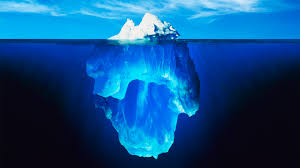Water’s (H2O) unique structure and very special unique properties also make it the perfect medium not only to support life, but to propagate waves. These properties include its density, its incompressibility, its surface tension, its odd freezing properties, and that it is a liquid under natural conditional and not a gas. Without all these properties there would be no life on Earth. If water was not unique in that its solid form (ice) was lighter than its liquid form, the oceans would freeze from the bottom up and freeze up completely in no time. If it were not so in-compressible, the ocean currents would stop flowing and you do not want to know what that would have done to the weather patterns and life.  Do you know what the pressure is like at the bottom of a 20’ deep pool? With over a mile of ocean water on top of it, water compresses less than 1%. If it were not for its surface tension, our blood vessels would be too thin for our blood to reach most of our bodies, and plants would have to grow along the ground. And these are just some of water’s marvelous properties.When we think of water and waves, we picture rolling ocean waves crashing at the shore or silvery pond waves rippling over the surface. Our bodies are 80% water, and whether we realize it or not, we are constantly bombarded with waves of all sorts of frequencies: some from far off in other galaxies; some from the earth; a lot from the Sun; many from the electrical circuity in our appliances, homes, and transmission lines; and all the time from cell towers and Wi-Fi transmitters trying to reach your cellphone and smart devices.  If it was not for the water in our bodies, we would not be able to withstand this constant onslaught.

It begs the question again, what is a wave? And why is it so compatible to life-giving water?

The Torah gives us the answer in its second verse,”God’s spirit moved on the water’s surface.”

What moves on the water’s surface? Waves.

“The water (המים)” is which word in the Torah? The 21st, as in the Name of G-d, associated with Binah, Ehyeh (אהיה), the place the 4 Forces and 4 Movements emanate from. It is also the 77th letter in the Torah, connecting it to mazal, another G-d given force, but also to the first 8 words of the Torah that comprise the 11 Triplets, one of the 4 Essential Elements of the Universe, analogous to the 4 Forces cited above.  The sum of those 8 words (33 letters) is 3003, which in turn is the sum of all the integers from 1 – 77.

The value 77 is also that of the word Tower (מִגְדַּל), which has an ordinal value of 32. As in the verse full of secrets from Proverbs 18:10 “מִגְדַּל עֹז שֵׁם יְהוָה בּוֹ יָרוּץ צַדִּיק וְנִשְׂגָּב. The name of YHVH is a strong tower; The righteous runs into it and elevated.” What is the Tower in the YHVH (יהוה)? It is the Vav(ו).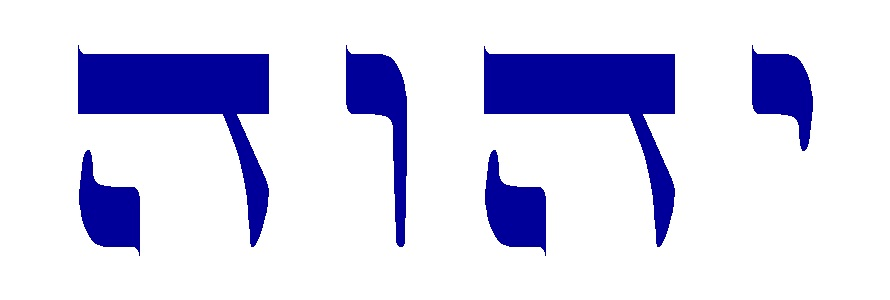Those 8 words bridge and link the first and second verses of the Torah, that begin with “In the Beginning (בראשית)” and end with “The water (המים).”

The phrase “In the Beginning water” has an ordinal value of 112, as in the 112 Triplets.

Earlier in the second verse it states, “The earth was without form and empty,” meaning there was no physicality; that was until G-d injected waves. ”God’s spirit moved on the water’s surface.” Then there could be light. The very next words are “God said, ‘There shall be light’” and physicists tell us light is a wave.

They also tell us it is a particle, or a particle that rides on a wave, or a wave and a particle at the same time, but not if you stop and look at it, then it changes.  At any rate, they still have a lot of figuring out to do to tell us why the sun shines, but they know it has something to do with waves.

Thank G-d for waves. Thank G-d for water. Thank G-d for Phi(φ). Thank G-d for the Hebrew Alef-bet. Imagine if we had to wait for man to invent them.

One final point. My vote for the Hebrew letter that the depicts the potentiality of the Wave field is the 21st letter, Shin (ש).

For anyone that has not been following this series from its inception, you can find the entire work here.

## 8 thoughts on “Part XVII Connecting the Dots Part 8”

1./\\/ says:

2020+1313=3333
3333/50= 66.66
3333 years since the reception of the Torah at Mt Sinai in 2448 HC 2448+3333= 5781 Hebrew year / 2020 Gregorian year
5780+2020= 7800
7/8= 0,875 -> 5780 backwards

Did you realised that you published this article on 07th of January 2020 – exactly 77 years since Tesla’s death (7 Jan 1943) and you mentioned his name and quotes him several times in it – on top of that 2020-1313= 707

2.Jeffrey Meiliken says:

Thank you. No, I had no idea. That is amazing synchronicity. And I spoke of the meaning of the number 77 multiple times too. None of what I wrote this section was planned ahead, nor was a date chosen; I just stopped writing and published it when it felt appropriate. Thank you again for pointing that out and your calculations.

3.Peter says:

Re אני The 72 Triplets and 61

We can regard the 36th and 37th Triplets as the central two Triplets of the 72 Triplets.

The combined value of their letters (94 and 61, “Ani”) is 155, which is te combined Atomic Numbers of the three Temple Metals, Copper, Silver and Gold, i.e. 29 + 47 + 79 = 155.

Interestingly, the numerical difference between 94 and 61 is 33, the number that “shows up” in the first 33 letters (first 8 words) or 11 Triplets, and in the dimensions of the Great Pyramid as 330 Cubits “as” 9075″ – 5775″ ==3300 inches for the difference between the Base (One Side) Length and Height of The Pyramid.

4.Peter says:

“The Water” and Some “Good Bottling”

Only an “idea”:

When the Torah was first given/dictated, it was as a string of letters. The letters that immediately follow המימ i.e. the first three letters of “Vayomer” at the start of Verse 3 are ויא of value 17, the value of tov/good, which is 17

Hamayim (the Water) at the end of verse 2 has gematria value 95

95 + 17 = 112 (Probably “intentional” as we jump from Water to Light via Waves/Speech)

5.Jeffrey Meiliken says:

Thanks Peter. I had noticed that, and it is no doubt of intentional design. Moreover, the first 3 initials of the 3rd verse are likewise ויא of value 17. These are the letters connecting “the waters” with “light.” The same 3 as in “There shall be light”

6.Jeffrey Meiliken says:

Very interesting. The three Temple Metals, Copper, Silver and Gold must play a profound and cryptic role or they would not be mentioned so often. For what its worth, the sum of their combined atomic weights = 368.380… and 368 is the value of the “And G-d said There shall be” Your two last comments are connected. Food for thought…

7.Peter says:

Spirit of God on The Waters

Just my mind “going sideways” with or from the notion (in this article) of what is in this article re רוח אלהימ and “The Waters” — i.e. the waves generating or imposing structure and form onto or into what was “formless”.

My mind went to the “Glassblower model” of נפש רוח הקודש נשמה but because of the “context” (Verse 2 of Genesis), I automatically substituted (in my head as I thought of the values/gematria) רוח אלהימ for רוח הקודש.

The “values” come out as 430 300 395 and 18 for חי

THAT IS OBVIOUSLY NOT THE “FULL FIVE” COMPONENTS IN THE GLASSBLOWER MODEL OF LEVELS OF SPIRIT, BUT, THOSE 4 LEVELS “SUM TO” 1143, WHICH IS THE VALUE OF THE TOP LINE OF THE 72 TRIPLETS.

Just a coincidence or maybe a “planned coincidence” ??

8.Peter says:

Still “tinkering with” the idea that the דמ in an אדמ, i.e. the blood in human blood vessels, will eventually be replaced with Light and/or Electromagenetic Energy. (In other words, the idea of Humans becoming “Fibre Optic” in the future).

presumably, if we still did or wanted to, eat, we would have Water in our systems somewhere/somehow. How Air (represented by the Aleph?) would fit into physiology that did not need Blood, is not as obvious.
(Presumably people would have given up smoking cigarettes if it meant that their eyeballs dimmed or slost their “light”?)

That covers the Shin and the Mem.